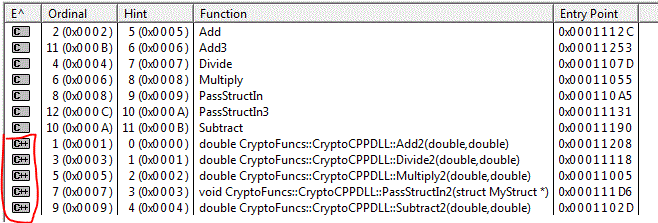## Thursday, 15 May 2014

### Creating C/C++ Dynamic Link Library (C/C++ DLL) Using Visual Studio

I was working on a project to create a C dynamic link library (.DLL). It seems difficult at the beginning. However, after several attempts, manage to produce it.

For creation of C++ library you can refer to the MSDN. I'm following these steps and enhance on the same codes to produce C DLL.

1. In order to access a library file, we can utilize the Dependency Walker.
2. The library that generated by the sample code by MSDN is as picture below. Take note of the difference between C++ functions and C functions.3. To export C functions, we can the export function names in .def file4. Header file will look something like this. The function for the Add is normal declaration in Header file.5. The implementation code is a standard code.
1. #include "stdafx.h"

2. #include "CryptoCPPDLL.h"
3. #include <stdexcept>

4. using namespace std;

5. namespace CryptoFuncs
6. {
7.     double CryptoCPPDLL::Add(double a, double b)
8.     {
9.         return a + b;
10.     }

11.     double CryptoCPPDLL::Subtract(double a, double b)
12.     {
13.         return a - b;
14.     }

15.     double CryptoCPPDLL::Multiply(double a, double b)
16.     {
17.         return a * b;
18.     }

19.     double CryptoCPPDLL::Divide(double a, double b)
20.     {
21.         if (b == 0)
22.         {
23.             throw invalid_argument("b cannot be zero!");
24.         }

25.         return a / b;
26.     }

27. double CryptoCPPDLL::Add2(double a, double b)
28.     {
29.         return a + b;
30.     }

31.     double CryptoCPPDLL::Subtract2(double a, double b)
32.     {
33.         return a - b;
34.     }

35.     double CryptoCPPDLL::Multiply2(double a, double b)
36.     {
37.         return a * b;
38.     }

39.     double CryptoCPPDLL::Divide2(double a, double b)
40.     {
41.         if (b == 0)
42.         {
43.             throw invalid_argument("b cannot be zero!");
44.         }

45.         return a / b;
46.     }
47. void CryptoCPPDLL::PassStructIn(MyStruct* myStruct)
48. {
49.       if (myStruct != NULL)
50.       {

51.             myStruct->SomeId = 234;
52.             myStruct->SomePrice = 456.23;
53.       }
54. }
55. void CryptoCPPDLL::PassStructIn2(MyStruct* myStruct)
56. {
57.       if (myStruct != NULL)
58.       {

59.             myStruct->SomeId = 234;
60.             myStruct->SomePrice = 456.23;
61.       }
62. }

63. void PassStructIn3(MyStruct* myStruct)
64. {
65.       if (myStruct != NULL)
66.       {

67.             myStruct->SomeId = 234;
68.             myStruct->SomePrice = 456.23;
69.       }
70. }

71. double Add3(double a, double b)
72. {
73. return a + b;
74. }

75. }
6. The second way to do this is through extern "C" __declspec(dllexport) YOUR_FUNCTION_NAME.
7. Hope this helps. I will create another post to how to do it in Eclipse using GCC.